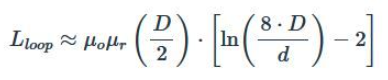cancel
Showing results for
Did you mean:
SOLVED

561 ViewsContributor II

In studying the instructional video 'NFC Antenna Design'

https://www.nxp.com/design/training/nfc-antenna-design:TIP-NFC-ANTENNA-DESIGN

In terms of the discussion about the determination of optimum radius of RFID reader antenna in relation to distance (10cm) from the tag antenna (at approximately 0:18:19 in the video) and the formula for the coupling coefficient, the mathematics appears to me to indicate that the optimum occurs when the radius is equal to the square root of 2 multiplied by their relative distance (a little over 14cm as opposed to 10cm as the author states).  Can anyone help me understand where I am misunderstanding the situation?  I simply set the derivative of the formula with respect to r to zero and solved for r.  See attached.  Thanks.

1 Solution
472 ViewsNXP TechSupport

Hello @tedj1 ,

Yes, you are correct with the mathematics.

The missing part is L0 of the reader ("L01"): the "single turn" inductance increases with the increasing r. That "reduces the coupling at higher r".

a simple formula is used like this:D = 2r = diameter of the antenna coil

d = wire diameter (= constant and small compared to D)

If you take this into account, the curve looks like plotted in the slides.

And in fact even in the curve result (as plotted in the slides), it can be seen that the maximum is not exactly at r=x, but slightly below... Still as a thumb rule it fits.

Have a great day,
Kan

-------------------------------------------------------------------------------
Note:
- We are following threads for 7 weeks after the last post, later replies are ignored
Please open a new thread and refer to the closed one, if you have a related question at a later point in time.
-------------------------------------------------------------------------------

2 Replies
473 ViewsNXP TechSupport

Hello @tedj1 ,

Yes, you are correct with the mathematics.

The missing part is L0 of the reader ("L01"): the "single turn" inductance increases with the increasing r. That "reduces the coupling at higher r".

a simple formula is used like this:D = 2r = diameter of the antenna coil

d = wire diameter (= constant and small compared to D)

If you take this into account, the curve looks like plotted in the slides.

And in fact even in the curve result (as plotted in the slides), it can be seen that the maximum is not exactly at r=x, but slightly below... Still as a thumb rule it fits.

Have a great day,
Kan

-------------------------------------------------------------------------------
Note:
- We are following threads for 7 weeks after the last post, later replies are ignored
Please open a new thread and refer to the closed one, if you have a related question at a later point in time.
-------------------------------------------------------------------------------

540 ViewsContributor II

I've linked my OpenOffice ods file worksheet, in case anyone is interested in checking my calculations.